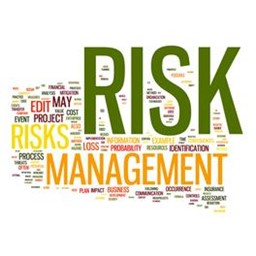22 Kasım 2014 - Cumartesi

# Probability & Statistics for Risk Modeling

İstanbul, Fatih#### Etkinlik Detayları

Objective:

Risk is an inherent factor of any business venture. Management of risk is an oft-overlooked facet of business; effective risk management is essential because it leads to stability and predictability, more efficient use of limited resources, and a higher chance of success. Effective risk management is reliant upon quantification through risk analysis and modeling via the mathematics of probability and statistics. In this one-day training course, we will cover basic probability & statistics as they relate to business risk modeling. The power and flexibility of Monte Carlo simulation modeling will be highlighted, and we will see practical application with several case studies. The included outline is subject to minor changes.

1. Risk & Risk Management
a. Motivating Example
b. Risk Modeling
c. Risk Management
2. Probability & Statistics
a. Uncertainty & Randomness
b. General rules for probabilities
c. Bayes Theorem
d. Probability Distribution Functions & Cumulative Distribution Functions
e. Dependent vs. independent variables
f. Descriptive statistics
3. Regression Models
a. Ordinary Least Squares regression
b. Multiple regression
c. Autoregression
4. Monte Carlo Simulation
a. Generating random variables from the Inverse CDF
b. Input distributions → Output distributions
c. Benefits of Monte Carlo simulation modeling
d. Common distributions for business risk modeling
5. Example Models (subject to change)
a. Example 1: Linear Cost-Volume-Profit Analysis
b. Example 2: Risk-based NPV Analysis
c. Example 3: Portfolio Value at Risk
d. Example 4: Probabilistic Risk Assessment
e. Example 5: Electric Power Plant Option Valuation

http://riskdynamicsconsultancy.com/egitimler/

22 Kasım 2014 Cumartesi 09:00

22 Kasım 2014 Cumartesi 17:00

TBD, İstanbul, Turkey, Fatih, İstanbul